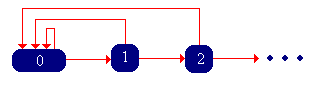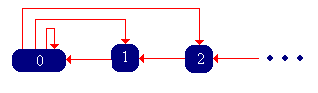]> Reliability Chains

## 8. Reliability Chains

#### The Success-Runs Chain

Suppose that we have a sequence of trials, each of which results in either success or failure. Our basic assumption is that if there have been $x$ consecutive successes, then the probability of success on the next trial is $p x$ where $p 0 1$. Whenever there is a failure, we start over, independently, with a new sequence of trials. Appropriately enough, $p$ is called the success function. Let $X n$ denote the length of the run of successes after $n$ trials.

Argue that $X X 0 X 1 X 2$ is a Markov chain with state space  and transition probability function

$P x x 1 p x , P x 0 1 p x , x$

This Markov chain is called the success-runs chain. The state graph of is given below:Now let $T$ denote the trial number of the first failure, starting with a fresh sequence of trials. Note that in the context of the success-runs chain $X$, $T T 0$, the first return time to state 0, starting in 0. Note that $T$ takes values in , since, presumably, it is possible that no failure occurs. Let $r n T n$ for $n$, the probability of at least $n$ consecutive successes, starting with a fresh set of trials. Let $f n T n$ for $n$, the probability of exactly $n 1$, consecutive successes, starting with a fresh set of trails.

Show that

1. $p x r x 1 r x$ for $x$
2. $r n x 0 n 1 p x$ for $n$
3. $f n 1 p n 1 x 0 n 2 p x$ for $n$
4. $r n 1 x 1 n f x$ for $n$
5. $f n r n 1 r n$ for $n$

Thus, the functions $p$, $r$, and $f$ give equivalent information. If we know one of the functions, we can construct the other two, and hence any of the functions can be used to define the success-runs chain.

The function $r$ is the reliability function associated with $T$. Show that it is characterized by the following properties:

1. $r$ is positive.
2. $r 0 1$
3. $r$ is strictly decreasing.

Show that the function $f$ is characterized by the following properties:

1. $f$ is positive.
2. $x 1 f x 1$

Essentially, $f$ is the probability density function of $T$, except that it may be defective in the sense that the sum of its values may be less than 1. The leftover probability, of course, is the probability that $T$. This is the critical consideration in the classification of the success-runs chain, which we consider in the next paragraph.

Verify that each of the following functions has the appropriate properties, and then find the other two functions:

1. $p$ is a constant in $0 1$. Thus, the trials are Bernoulli trials.
2. $r n 1 n 1$ for $n$.
3. $r n n 1 2 n 1$ for $n$.
4. $p x 1 x 2$ for $x$.

The success-runs applet is a simulation of the success-runs chain based on Bernoulli trials. Run the simulation 1000 times for various values of $p$, and note the limiting behavior of the chain.

#### Recurrence and the Remaining Life Chain

From the state graph, show that the success-runs chain is irreducible and aperiodic.

Recall that $T$ has the same distribution as the first return time to 0 starting at state 0. Thus, the classification of the chain as recurrent or transient depends on $α T$. Specifically, the success-runs chain is transient if $α 0$ and recurrent if $α 0$. Thus, we see that the chain is recurrent if and only if a failure is sure to occur. We can compute the parameter $α$ in terms of each of the three functions that define the chain.

Show that

$α x 0 p x n r n 1 x 1 f x$

When the success-runs chain $X$ is recurrent, we can define a related random process. Let $Y n$ denote the number of trials remaining until the next failure, after $n$ trials.

Argue that $Y Y 0 Y 1 Y 2$ is a Markov chain with state space  and transition probability function

$Q 0 x f x 1 , Q x 1 x 1 , x$

The Markov chain $Y$ is called the remaining life chain. Verify the state graph below and show that this chain is also irreducible, aperiodic, and recurrent.Compute $α$ and determine whether the success-runs chain $X$ is transient or recurrent for each of the cases in Exercise 5.

Run the simulation of the success-runs chain 1000 times for various values of $p$, starting in state 0. Note the return times to state 0.

#### Positive Recurrence and Limiting Distributions

Let $μ T$, the expected trial number of the first failure, starting with a fresh sequence of trials.

Show that the success-runs chain $X$ is positive recurrent if and only if $μ$, in which case the invariant distribution has probability density function $g$ given by

$g x r x μ , x$

Show that

1. If $α 0$ then $μ$
2. If $α 0$ then $μ n 1 n f n$
3. $μ n 0 r n$

Suppose that $α 0$, so that the remaining life chain $Y$ is well-defined. Show that this chain is also positive recurrent if and only if $μ$, with the same invariant distribution as $X$ (with probability density function $g$ given in the previous exercise).

Determine whether the success-runs chain $X$ is transient, null recurrent, or positive recurrent for each of the cases in Exercise 5. If the chain is positive recurrent, find the invariant probability density function.

The success-runs chain corresponding to Bernoulli trials has a geometric distribution as the invariant distribution. Run the simulation of the success-runs chain 1000 times for various values of $p$. Note the apparent convergence of the empirical distribution to the invariant distribution.

#### Time Reversal

Suppose that $μ$, so that the success-runs chain $X$ and the remaining-life chain $Y$ are positive recurrent.

Show that $X$ and $Y$ are time reversals of each other, and use this fact to show again that $g$ is the invariant probability density function.

Run the simulation of the success-runs chain 1000 times for various values of $p$, starting in state 0. If you imagine watching the simulation backwards in time, then you can see a simulation of the remaining life chain.